# 35 math problems for 5th gradersAuthor
Amber WatkinsPublished
September 13, 2023# 35 math problems for 5th gradersAuthor
Amber WatkinsPublished
September 13, 2023# 35 math problems for 5th gradersAuthor
Amber WatkinsPublished
September 13, 2023Key takeaways

• 5th-grade math word problems help students apply mathematical concepts to real-world scenarios.
• In fifth-grade math, it’s important to master fractions, decimals, and order of operations.
• Hard problems for 5th graders can be simplified by drawing a picture or using a method that makes them easier to solve.

If this math page has 5 sections of math problems for 5th graders with an average of 7 questions in each section, can you guess how many problems there will be in all? That’s right, there will be a total of 35 math problems for 5th graders for us to practice.

Without realizing it, you just solved one of our first 5th-grade math word problems. Excellent work!

Together we will review fifth-grade math problems with decimals, fractions, order of operations, and rounding. We will even consider hard math problems for 5th graders, like multiplying using square models and number lines. Don’t worry about getting the answers right the first time, each section includes math problems for 5th graders with answers if you need a sneak peek.

So grab a pad and pencil and let’s begin.

## Word problems for 5th gradersLet’s begin by covering math word problems for 5th graders.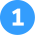Mia’s water bottle can hold 24 ounces of water. She drinks two full water bottles each day. How many total cups of water does Mia drink each day? [Conversions]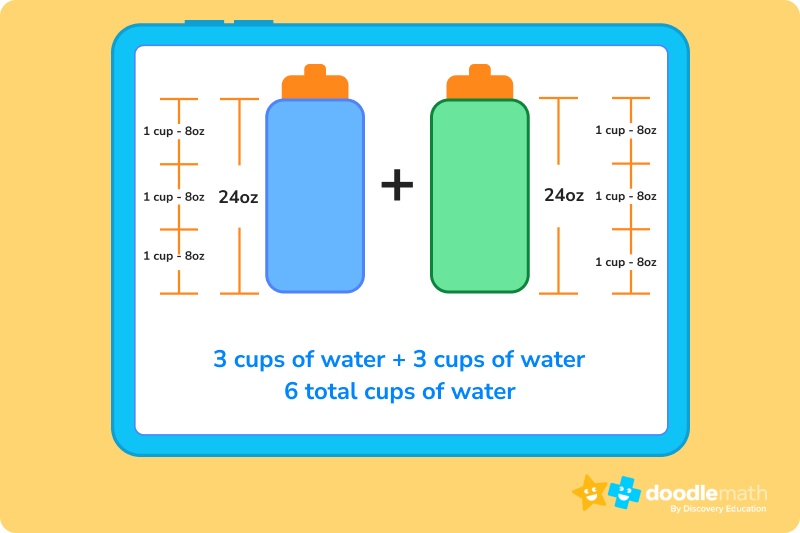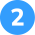The playground is made up of four rectangular lots that are each 10 ft by 7 ft. What is the total area of the playground? [Finding area]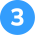Josh lives 2.5 miles away from the park. Josh rides his bike to the park and back home four days a week. How many miles does Josh ride his bike each week? [Calculations]

Top tip!

Since we are solving word problems, a word should always be in our answer.

## Writing numbersIn this section, we will cover math problems for 5th graders that use place values, word form to standard form, standard form to word form, and expanded form.Which digit in the number 245 is in the hundreds place?Which digit in the number 4,602 is in the tens place?Which digit in the number 752 is in the ones place?

## 5th Grade math problems - standard form to expanded form

4,000 + 300 + 2 = 4,302

600 + 50 + 4 = 654

20,000 + 2,000 +300 + 60 + 5 = 22,365

## Using Decimals

In this section, we will cover fifth-grade math problems that include comparing decimals and rounding decimals to the nearest tenth, hundredth, and thousandths place. Let’s get started.

8.9 and 8.900 are the same.

Top tip!

Remember if the number to the right is 5 or larger, you can borrow and become one digit larger. If the number to the right is between 0 and 4, you can’t borrow and the number remains the same.

## ArithmeticIn this section, we will review math problems for fifth graders that cover the topics of adding and subtracting two and three-digit numbers using transformation, division using area models, and the order of operations.

### 5th Grade Math Practice: adding and subtracting whole numbers by transformation

A large portion of fifth-grade math problems can be made easier to solve by transformation or changing numbers to make the problem simpler. Let’s practice adding and subtracting two and three-digit numbers by transformation.

How to add numbers using transformation?

When adding or subtracting numbers, look for ways to round to make the problem simpler. Don’t forget what you do to one number, you have to do the opposite to the other. This makes sure the problem stays balanced. Let’s see how this is done.

What is 48 + 52?

48 + 2 = 50

52 – 2 = 50

50 + 50 = 100.

It may be difficult to add 48 + 52 in your head, so we round 48 to 50 by adding 2. Since we added 2 to the first number, we have to make sure to subtract 2 from the second number. So now the problem is 50 plus 50, which is much easier to calculate!

32 – 2 = 30
68 + 2 = 70
30 + 70 = 100

208 – 8 = 200
432 + 8 = 440
200 + 440 = 640

321- 1 = 320
199 + 1 = 200
320- 200 = 120## 5th grade math problems - division using area modelsDon’t know what an area model is? See our area model guide to help with these problems.Using an area model find the quotient of 365 ፥ 5 =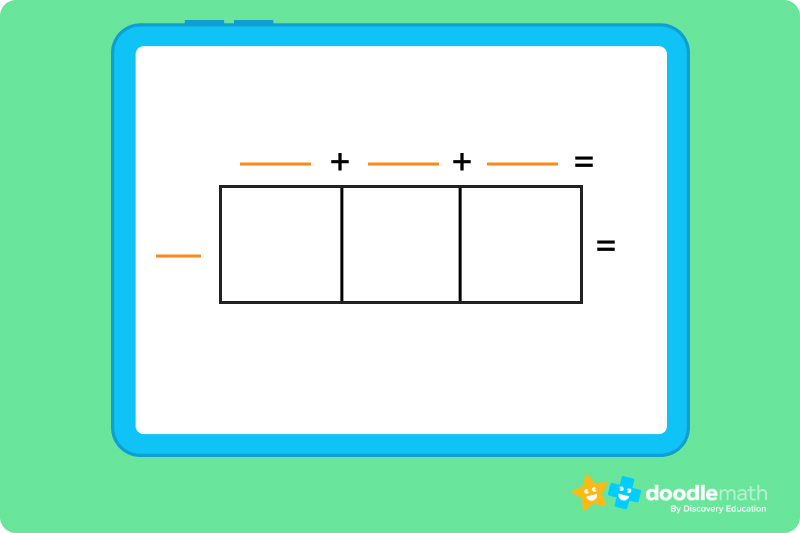Using an area model find the quotient of 504 ፥ 6 =Using the area model below, find the quotient of 872 ፥ 2 =## 5th Grade math problems - order of operations

Many people remember the Order of Operations with the acronym PEMDAS- “Please Excuse My Dear Aunt Sally”, with each letter representing an operation: Parentheses, Exponents, Multiply or Divide, Add or Subtract. Solve the following equations using the order of operations.

Top tip!

Remember that the steps Multiply or Divide can be done in any order. Also, the steps Add or Subtract can be done in any order, do the operation that comes first.

## 5th grade math problems - hard math problems for 5th gradersSome of the hardest math problems for fifth graders involve multiplying: multiplying using square models, multiplying fractions and whole numbers using expanded form, and multiplying fractions using number lines. Let’s practice a few of each!

### 5th grade math practice - multiplying using square models

Multiplying using square models is a method to help students “see” multiplication problems in a simpler way.Multiply 42 x 35 using the square model below.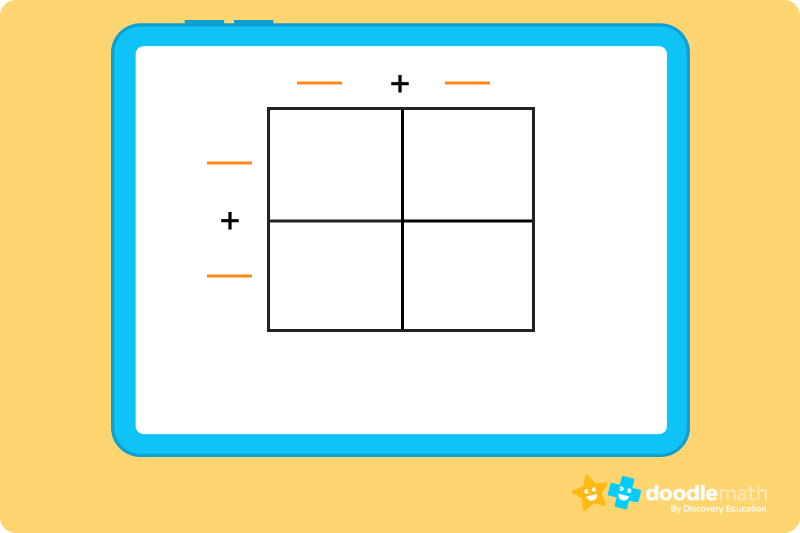Multiply 55 x 76 using the square model below.Multiply 98 x 42 using the square model below.### 5th grade math practice - multiplying fractions and whole numbers using expanded formMultiply ½ x 5 using expanded form.Multiply ⅔ x 6 using expanded form.Multiply ⅙ x 7 using expanded form.

### 5th grade math practice - multiplying fractions using a number lineMultiply ½ x 4/6 by using a number line.Mia drinks 6 cups of water each day. Explanation: There are 8 ounces in each cup. So 24 divided by 8 is equal to 3 cups each day. [Insert image ex below]280 square feet. Explanation: The area of one lot is 10 x 7 = 70 square feet. Take that area and times it by four, so 70 x 4 = 280 square feet.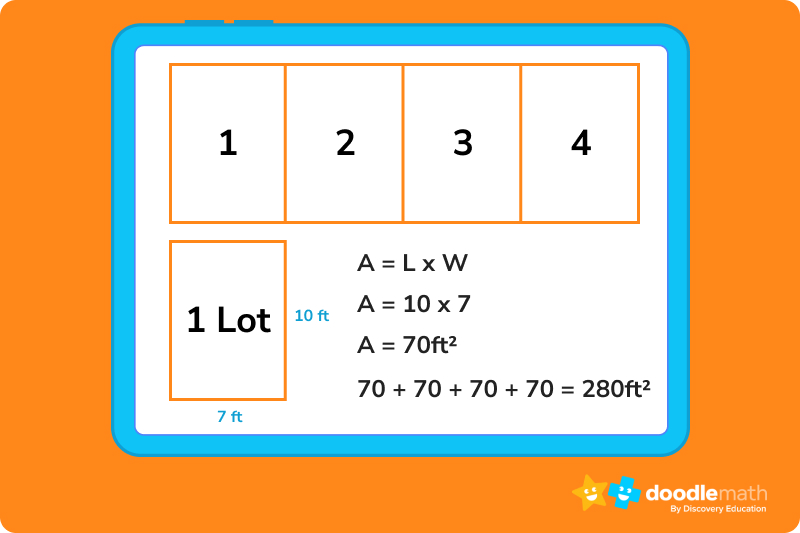Josh rides 20 miles on his bike each week. Explanation: Each day Josh rides 5 total miles: 2.5 miles to the park and 2.5 miles back home. If we multiply that number by 4 days a week, it equals 20 miles in all.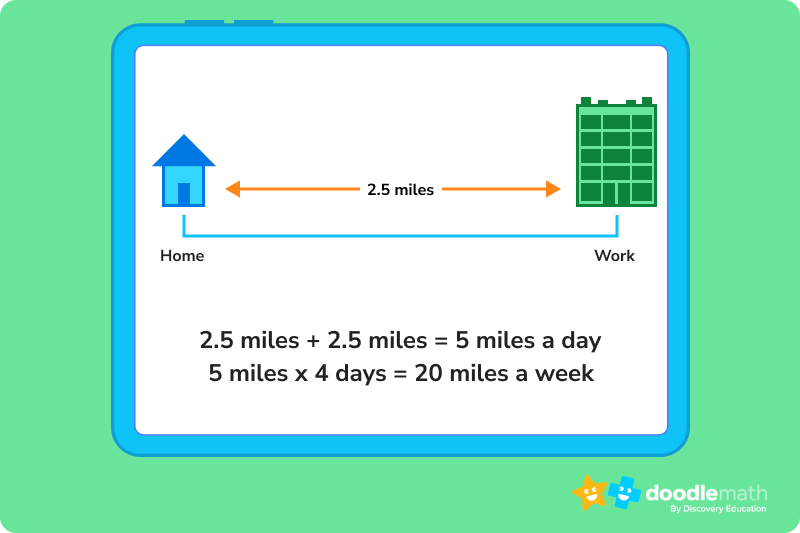2 is in the hundreds place.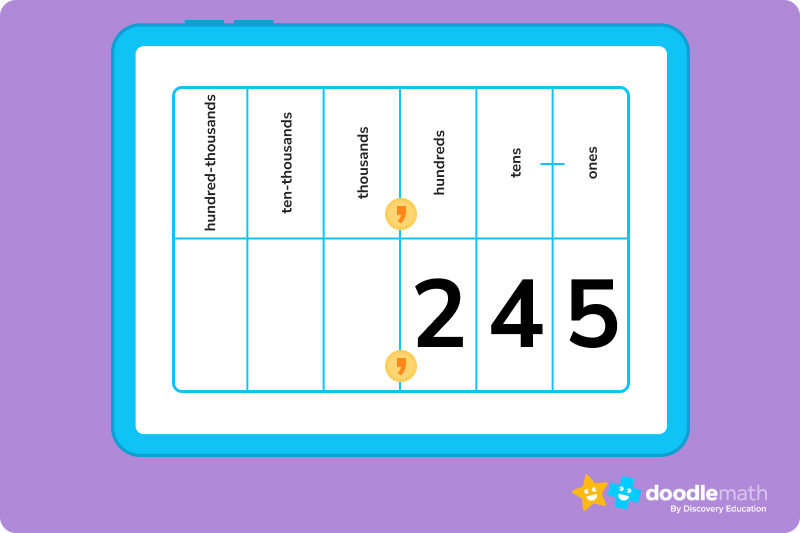0 is in the tens place.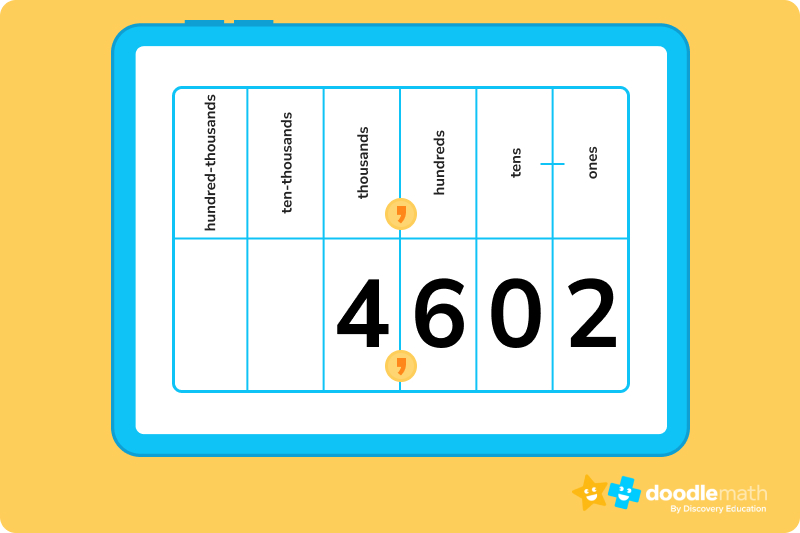2 is in the ones place.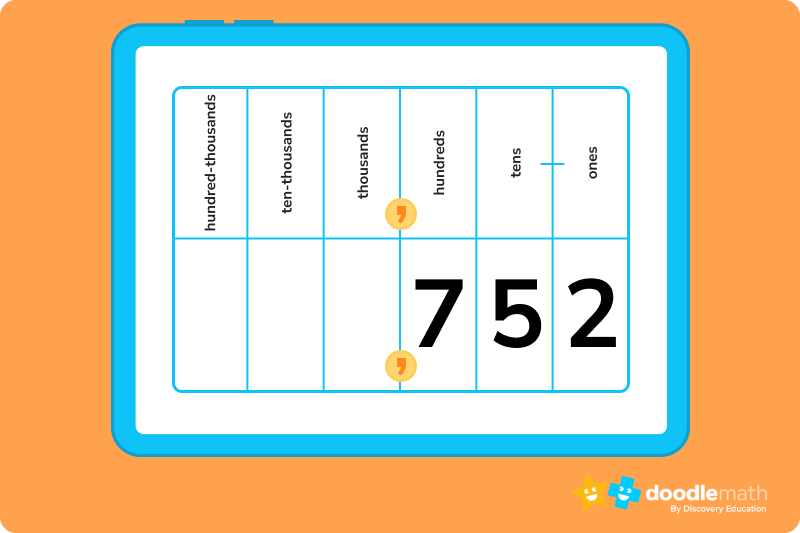## Division using area models answer sheet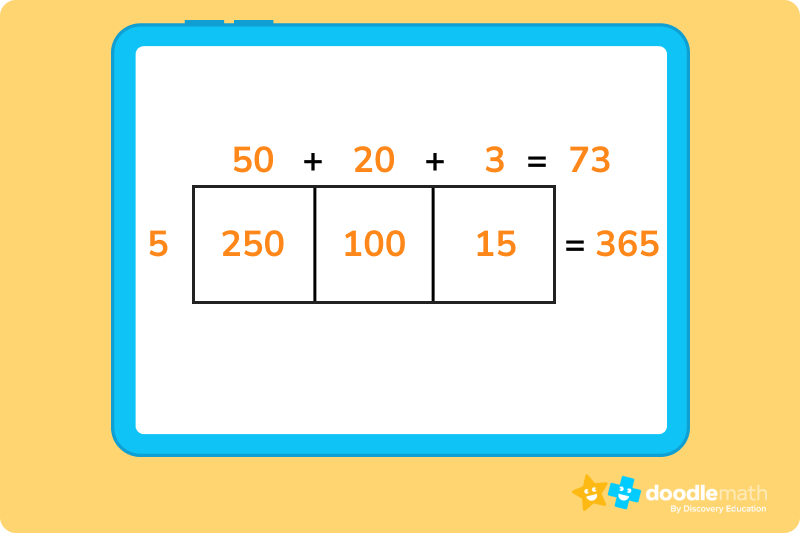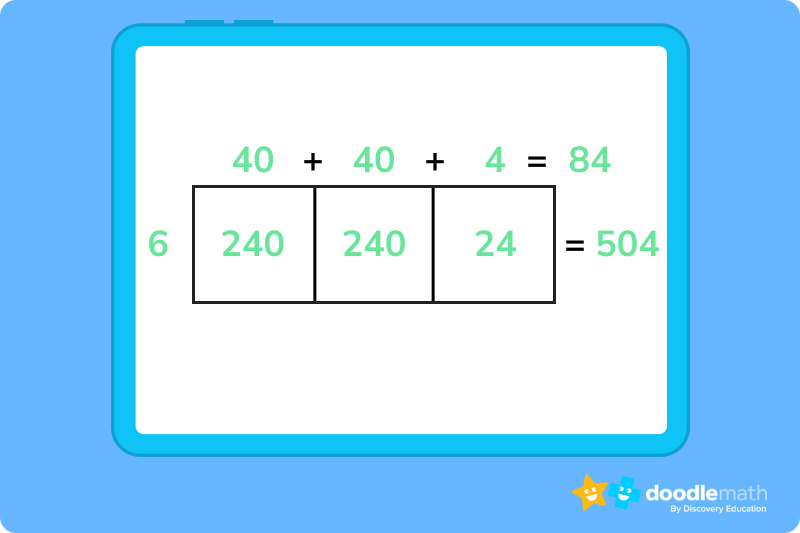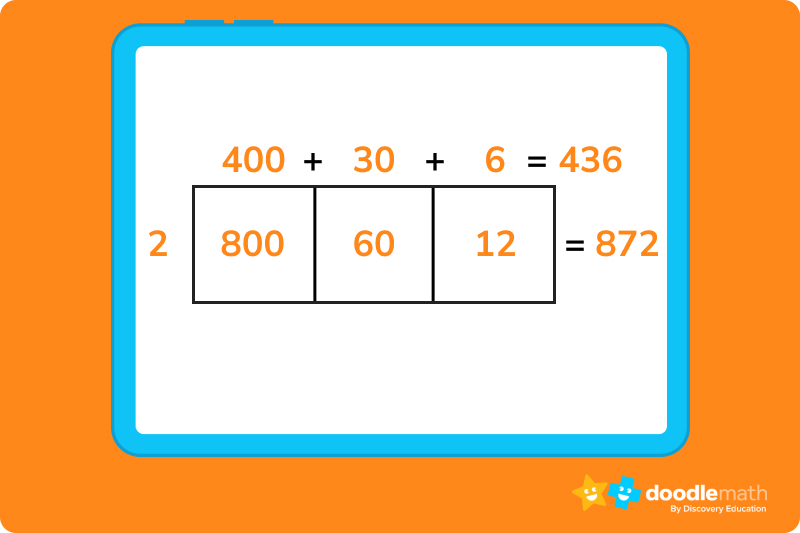## Multiplying using square models answer sheet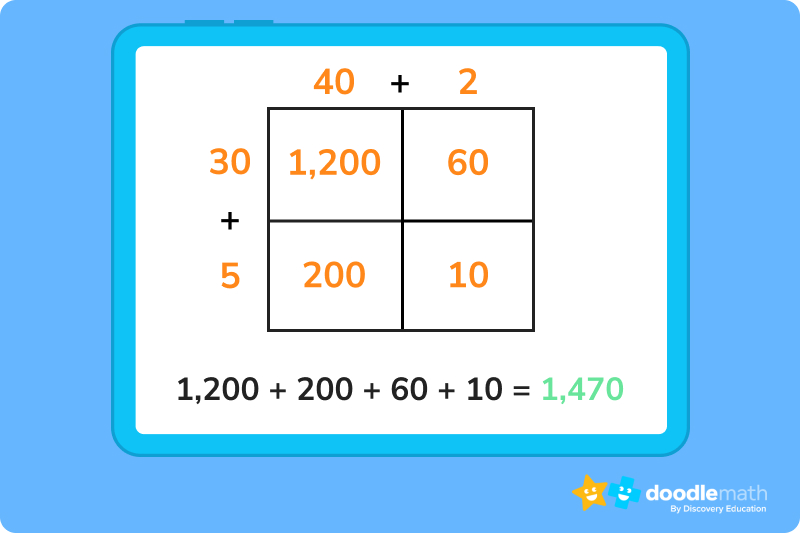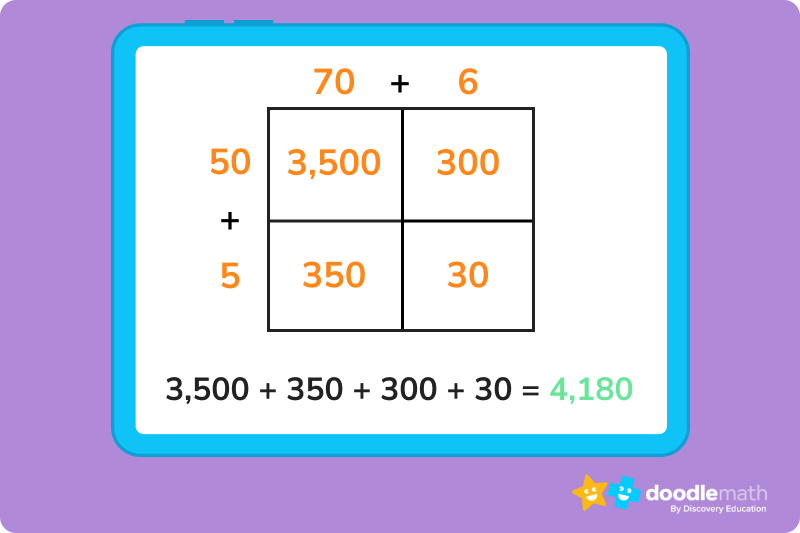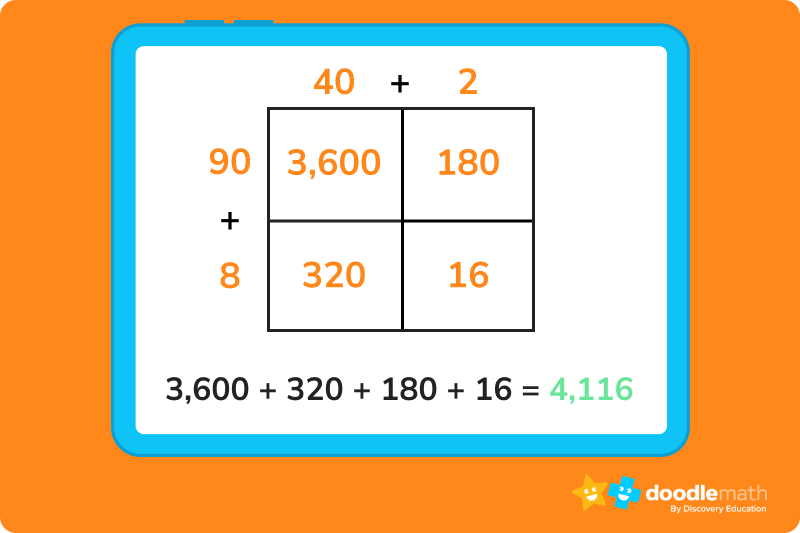## Multiplying fractions and whole numbers using expanded form answer sheet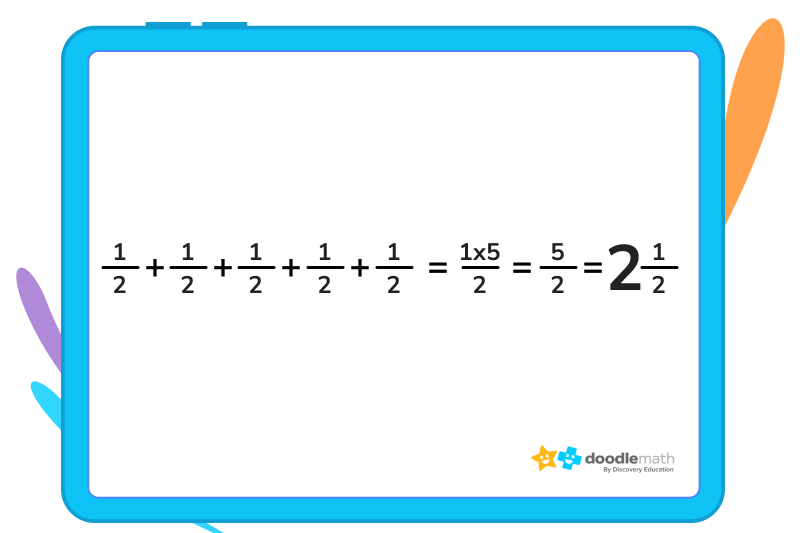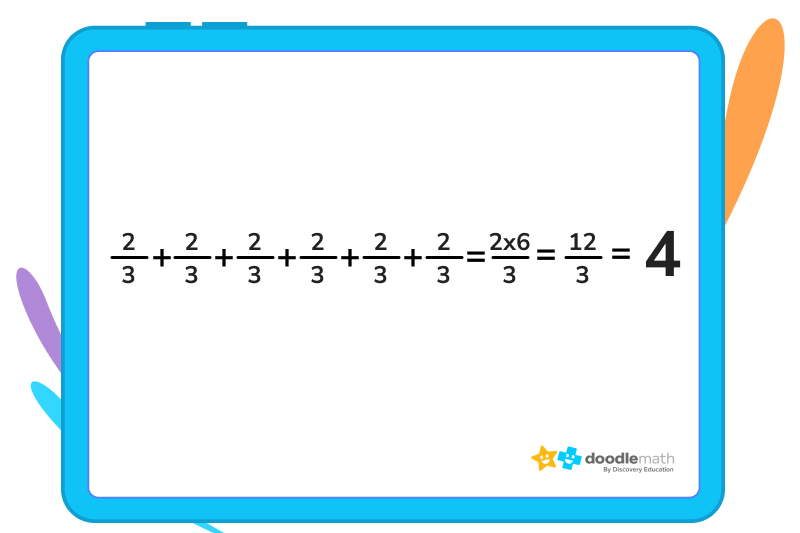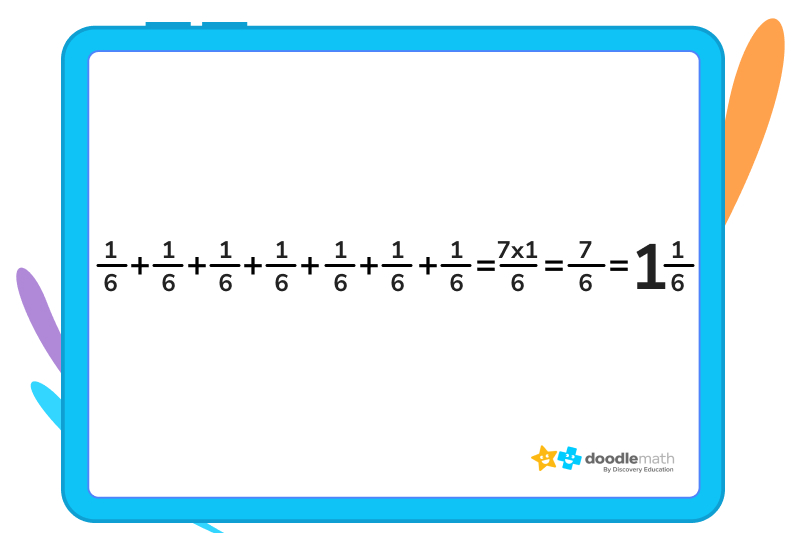## Multiplying fractions using a number line answer sheet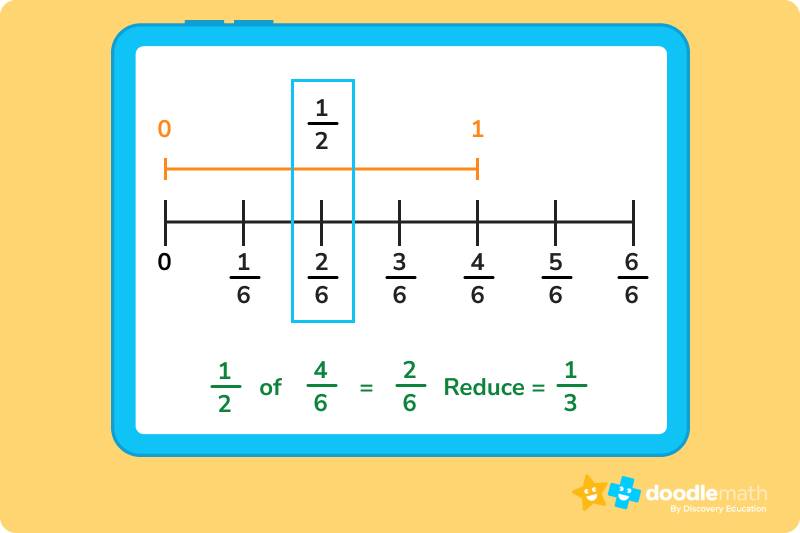Explanation – First divide the number line into 6 equal parts and draw up a line up to 4/6. Then find the ½ way mark of that line you drew up to 4/6. You will now see the line is divided into three parts. So the answer would be ⅓.

For more math practice for 5th graders, our app for math help provides 5th-grade math help in a way that makes math fun, rewards effort over being correct, and scaffolds learning. Plus, you’ll get access to a dashboard that displays your child’s participation and progression on math topics they struggle with. Check it out today!

Lesson credits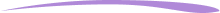Amber Watkins

Amber is an education specialist with a degree in Early Childhood Education. She has over 12 years of experience teaching and tutoring elementary through college level math. "Knowing that my work in math education makes such an impact leaves me with an indescribable feeling of pride and joy!"

Amber Watkins

Amber is an education specialist with a degree in Early Childhood Education. She has over 12 years of experience teaching and tutoring elementary through college level math. "Knowing that my work in math education makes such an impact leaves me with an indescribable feeling of pride and joy!"

# Are you a parent, teacher or student?

Are you a parent or teacher?

## Hi there!

Book a chat with our team